# NMAT Quantitative Skills MCQ Quiz - 1

## 48 Questions MCQ Test NMAT Mock Test Series | NMAT Quantitative Skills MCQ Quiz - 1

Description
Attempt NMAT Quantitative Skills MCQ Quiz - 1 | 48 questions in 60 minutes | Mock test for CAT preparation | Free important questions MCQ to study NMAT Mock Test Series for CAT Exam | Download free PDF with solutions
QUESTION: 1

### Group Question Answer the following question based on the information given below. In an MBA entrance exam, questions are classified into two sections - Quants and Verbal. The number of questions in Quants is less than 100 while that in Verbal is not less than 75. Within each section, the questions are classified into Easy or Difficult based on the number of concepts involved. An easy question requires not more than 1 concept, while a difficult question requires 2 or more concepts to solve.Further, a question could be lengthy (atleast 3 minutes to solve) or non- lengthy (less thanl minute to solve). Based on the following data, answer the subsequent questions. 1. The number of difficult, non-lengthy questions in Verbal is 10 less than half the number of easy, non-lengthy questions in Quants. 2. The ratio of number of easy, lengthy questions in Quants to number of difficult, lengthy questions in Verbal is 5:3. 3. The number of non-lengthy, difficult questions in Quants is 5 less than thrice the number of lengthy, easy questions in Verbal. 4. The number of lengthy questions in Verbal is 35 less than the number of easy questions in Quant. 5. The number of non-lengthy questions in Quants is 30 more than the number of difficult questions in Verbal. 6. The number of easy, non lengthy question in Verbal is thrice the number of lengthy, difficult questions in Quant. 7. Each sub-section had atleast 5 questions.   Q.What is the number of easy, but lengthy questions in the Verbal section?

Solution:

Represent Quant-lengthy-easy as Q-L-E, Verbal-non-lengthy-difficult as V-NL-D and so on.
Let Q-L-E = 5x, Q-NL-E = 2z, Q-L-D = K and V-L-E = y

V-L-D = 3x (from 2), V-NL-D = z - 10 (from 1), V-NL-E = 3K (from 6) and Q-NL-D = 3y - 5 (from 3)

Now, the questions in Quants and Verbal can be classified as easy/difficult or lengthy/non-lengthy as shown below.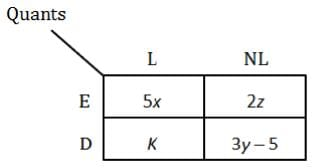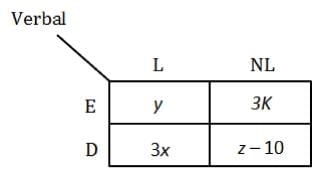The number of non-lengthy questions in Quants is 30 more than the number of difficult questions in Verbal.

2z + 3y - 5 = (3x + z — 10) + 30

3y + z - 3x = 15 ...(i)

The number of lengthy questions in Verbal section is 35 less than the number of easy questions in Quant.

y + 3x = (5x + 2z) - 35

2x - y + 2z = 35 ...(ii)

From (i) and (ii)

7y - 8x = 15

(x,y) = (6, 9) or (13, 17) or (20, 25) etc.

Corresponding value for z = 16 or 13 or 10 etc..
Since each sub-section has at least 5 qestions, the number of non-lengthy, difficult question in the verbal section
(z - 10) > 5.
> 15
z = 16
Thus, (x,y ,z) = (6, 9, 16) Also,the number of question in Quants is less than 100 while that in verbal is not less than 75.
Number o f questions in Quants = 5x + 2z + (3y - 5 ) + K = M + K < 100

QUESTION: 2

### In an MBA entrance exam, questions are classified into two sections - Quants and Verbal. The number of questions in Quants is less than 100 while that in Verbal is not less than 75. Within each section, the questions are classified into Easy or Difficult based on the number of concepts involved. An easy question requires not more than 1 concept, while a difficult question requires 2 or more concepts to solve.Further, a question could be lengthy (atleast 3 minutes to solve) or non- lengthy (less thanl minute to solve). Based on the following data, answer the subsequent questions. 1. The number of difficult, non-lengthy questions in Verbal is 10 less than half the number of easy, non-lengthy questions in Quants. 2. The ratio of number of easy, lengthy questions in Quants to number of difficult, lengthy questions in Verbal is 5:3. 3. The number of non-lengthy, difficult questions in Quants is 5 less than thrice the number of lengthy, easy questions in Verbal. 4. The number of lengthy questions in Verbal is 35 less than the number of easy questions in Quant. 5. The number of non-lengthy questions in Quants is 30 more than the number of difficult questions in Verbal. 6. The number of easy, non lengthy question in Verbal is thrice the number of lengthy, difficult questions in Quant. 7. Each sub-section had atleast 5 questions.   Q.Which sub-section has the minimum number of questions?

Solution:

Consider the solution to first question. Number of questions in each subsection given in the options is: Option 1: V-L-E =y = 9

Option 2: Q-NL-D = 3y - 5 = 3(9) - 5 = 22

Option 3: V-NL-E = 3K= 3(14) or 3(15) = 42 or 45

Option 4: Q-L-D = K= 14 or 15
Option 5: V-NL-D = z - 10 = 16 - 10 = 6 Hence, option 5.

QUESTION: 3

### In an MBA entrance exam, questions are classified into two sections - Quants and Verbal. The number of questions in Quants is less than 100 while that in Verbal is not less than 75. Within each section, the questions are classified into Easy or Difficult based on the number of concepts involved. An easy question requires not more than 1 concept, while a difficult question requires 2 or more concepts to solve.Further, a question could be lengthy (atleast 3 minutes to solve) or non- lengthy (less thanl minute to solve). Based on the following data, answer the subsequent questions. 1. The number of difficult, non-lengthy questions in Verbal is 10 less than half the number of easy, non-lengthy questions in Quants. 2. The ratio of number of easy, lengthy questions in Quants to number of difficult, lengthy questions in Verbal is 5:3. 3. The number of non-lengthy, difficult questions in Quants is 5 less than thrice the number of lengthy, easy questions in Verbal. 4. The number of lengthy questions in Verbal is 35 less than the number of easy questions in Quant. 5. The number of non-lengthy questions in Quants is 30 more than the number of difficult questions in Verbal. 6. The number of easy, non lengthy question in Verbal is thrice the number of lengthy, difficult questions in Quant. 7. Each sub-section had atleast 5 questions.   Q.Which among the following could be a possible value of total number of easy questions?

Solution:

Consider the solution to the first question.

Number of easy questions = 5x + 2z + y + 3K

(x,y,z) = (6, 9, 16)

Number of easy questions = 71 + 3K K= 14 or 15

Number of easy questions =113 or 116 Hence, option 1.

QUESTION: 4

In an MBA entrance exam, questions are classified into two sections - Quants and Verbal. The number of questions in Quants is less than 100 while that in Verbal is not less than 75. Within each section, the questions are classified into Easy or Difficult based on the number of concepts involved. An easy question requires not more than 1 concept, while a difficult question requires 2 or more concepts to solve.Further, a question could be lengthy (atleast 3 minutes to solve) or non- lengthy (less thanl minute to solve).

Based on the following data, answer the subsequent questions.

1. The number of difficult, non-lengthy questions in Verbal is 10 less than half the number of easy, non-lengthy questions in Quants.

2. The ratio of number of easy, lengthy questions in Quants to number of difficult, lengthy questions in Verbal is 5:3.

3. The number of non-lengthy, difficult questions in Quants is 5 less than thrice the number of lengthy, easy questions in Verbal.

4. The number of lengthy questions in Verbal is 35 less than the number of easy questions in Quant.

5. The number of non-lengthy questions in Quants is 30 more than the number of difficult questions in Verbal.

6. The number of easy, non lengthy question in Verbal is thrice the number of lengthy, difficult questions in Quant.

7. Each sub-section had atleast 5 questions.

Q.Find the maximum possible score of the topper, if each correct option fetches 4 marks?

Solution:

Consider the solution to the first question.
Total questions = 5x + 2z + K + 3 y ~ 5 + y + 3K +3 x + z - 10

= 8x + 4y + 3z + 4K - 15

(x,y,z) = (6, 9, 16)

Total questions = 8(6) + 4(9) + 3(16) + 4AT— 15 = 117 + 4K

K= 14 or 15

Maximum number of questions =117 +(4 x 15) =177

The score is maximized if the topper answers each question correctly.
Maximum score = 177 x 4 = 708 Hence, option 5.

QUESTION: 5

Which value of x does satisfy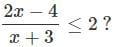Solution: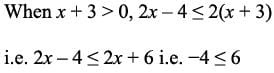Since this is always true, x + 3 > 0 i.e. x > -3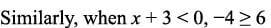Since this is never true, x + 3 is not less than 0.
Except for x = -4, all the other values satisfy x > -3.
Hence, option 3.

QUESTION: 6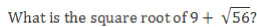Solution: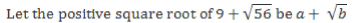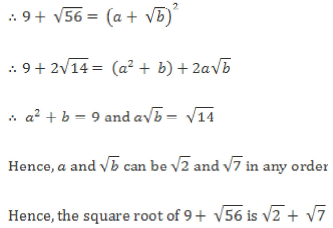Hence, option 3.

QUESTION: 7

What is the largest of three consecutive natural numbers if the cube of the first number is smaller than their product by 5, the cube of the second number is greater than their product by 2 and the square of the third number exceeds their product by 3.

Solution:

The largest number cannot be 1 or 2 as is the largest of three consecutive natural numbers.
Now, consider the options.
If the largest number is 3, the numbers are 1,2 and 3. Their product is 6. It exceeds the cube of 1 by 5, is less than the cube of 2 by 2 and is less than the square of 3 by 3.
Thus 3 satisfies all the conditions.
Hence, option 1.

QUESTION: 8

From a block of ice cream in the shape of a cuboid of length, breadth and height as a, b, c units respectively, two scoops of ice cream are taken out and served per person. The scoop is in the shape of a hemisphere of radius R. If there is no wastage, how many people get the ice cream?

Solution:

Total volume of the block of ice-cream = (Volume of the hemispherical scoop) x 2 x (no. of people served)

Let x people get the ice cream.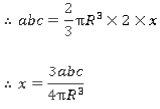Hence, option 2.

QUESTION: 9

If Rohan was born on 29th March 1990 on Thursday, on what day on the week will his 25th birthday fall?

Solution:

Rohan’s 25th birthday will be on 29th March 2015.
Now, the first 2000 completed years comprise 5 sets of 400 years each. Since there are 0 odd days in 400 years, there are 0 odd days in the first 2000 years as well.
The 14 completed years from 2000 to 2014 have 3 leap years (2004, 2008 and 2012) and 11 non-leap years.
Since a non-leap year has 1 odd day and a leap year has 2 odd days,

Total odd days in these 14 years = 11(1) + 3(2) = 17 = 7(2) + 3 i.e. 3 odd days.

Total number of days in 2015 (upto 29th March) = 31 + 28 + 29 = 88 days (as 2015 is not a leap year).
Number of odd days in this period = 7(12) + 4 i.e. 4 odd days.
So, total odd days till Rohan’s birthday = 3 + 4 = 7 i.e. 0 odd days.
Hence, Rohan’s 25th birthday will fall on a Sunday.
Hence, option 3.

QUESTION: 10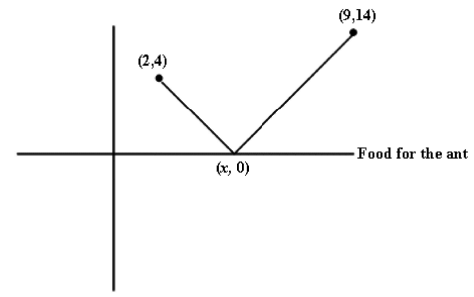An Ant is at (2, 4) and wants to go to (9, 14) but in the process it has to carry food lying on the x-axis. Where should it touch the x-axis, so that it has to cover minimum distance in the entire journey?

Solution:

If we try to solve this problem by using distance formula, it will require a lot of calculations.
Let us approach this problem in a different way.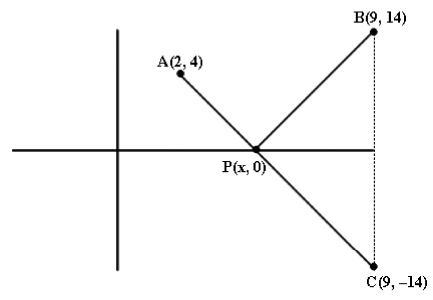‘C’ is the mirror image of B(9, 14)

Now the path taken by the ant is APB, where P(x, 0).
We know that PE = PC

We can safely say that path taken by the ant is APC.
And we need to find minimum length of APC.
APC will be minimum if A, P, C lie on a same straight line,

i.e. AC = AP + PC

Equation of line AC is: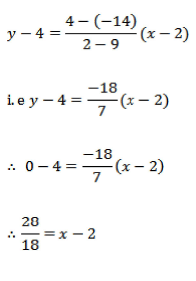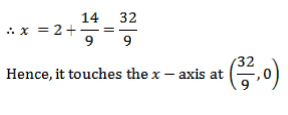Hence, option 4.

QUESTION: 11

Group Question

Answer the following question based on the information given below.

The first pie chart below shows the percentage distribution of three components the weight of a human being while the second pie chart shows the percentage distribution of protein (in terms of weight) across different body parts.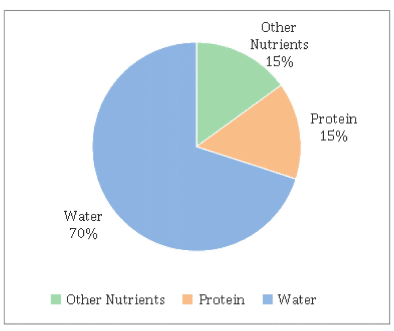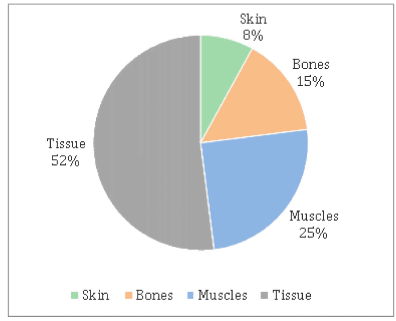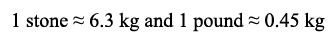Q.If Zebesco, a professional wrestler, weighs 21 stone, how much of his body weight (in pounds) does not come from protein in tissue?

Solution:

Weight of Zebesco that does not come from protein in tissue = 21 -1.638 = 19.362 stone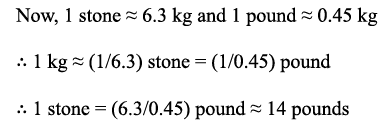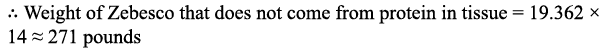Hence, option 3.

QUESTION: 12

The first pie chart below shows the percentage distribution of three components the weight of a human being while the second pie chart shows the percentage distribution of protein (in terms of weight) across different body parts.Q.‘Other nutrients’ in the body comprises minerals, carbohydrates, fats and vitamins in the ratio 2 : 4 : 1 : 3. What is the maximum possible difference (in stone) between the weight contributed by protein in skin and fats for a gymnast weighing 52 kg?

Solution:

Weight contributed by protein in skin = 0.08 x 0.15 x 52 = 0.624 kg

The difference between this value and the weight contributed by fat can be maximized when fat contributes the least to ‘other nutrients’.

Weight contributed by fat = (1/10) x 0.15 x 52 = 0.78 kg

Maximum difference = 0.78 - 0.624 = 0.156 kg = (0.156/6.3) stone ~ 0.02 stone

Hence, option 1.

QUESTION: 13

The first pie chart below shows the percentage distribution of three components the weight of a human being while the second pie chart shows the percentage distribution of protein (in terms of weight) across different body parts.Q.Gaurav weighs 65 kg. What percentage of his body weight comes from muscles?

Solution:

The weight due to protein in muscles can be found. However, it is not known if water or other nutrients also contribute to muscle weight, and if so, in what proportion.
Hence, the body weight due to muscles cannot be found.
Hence, option 5.

QUESTION: 14

The first pie chart below shows the percentage distribution of three components the weight of a human being while the second pie chart shows the percentage distribution of protein (in terms of weight) across different body parts.Q.Which of these is the heaviest component?

Solution: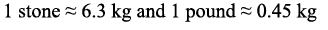1 stone > 1 kg > 1 pound

Also 1 stone =14 pounds

Convert all the weights given into a common unit, say stone.
Option 1: 52% of 15% of 13.5 stone Option 2: 40% of 15% of some amount less than 10 stone (because 10 stone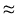kg)

Option 3: 70% of 10.3 stone

Option 4: 30% o f some amount less than 9 stone (because 9 stone = 9 x 1 4 pounds =126 pounds)

Option 5: 23% of 15% of some amount less than 8 stone (as explained in option 4).
By observation itself, options 2, 4 and 5 will be less than option 3.
Also, option 3 is nearly 7 stone while option 1 is not even 2 stone.
Hence, the weight in option 3 is the greatest.
Hence, option 3.

QUESTION: 15

Group Question

Answer the following question based on the information given below.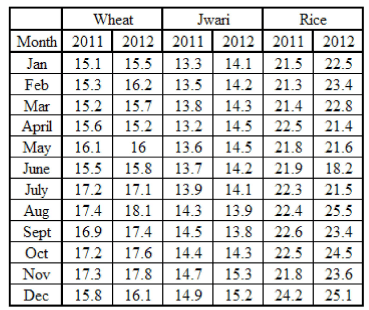Cereal consumption of Maharashtra state in 000 ton

Q.What is the approximate ratio of the difference between the maximum and minimum monthly wheat consumption for 2011 and the difference between the maximum and minimum monthly rice consumption for 2012?

Solution:

Minimum monthly wheat consumption in 2011 = 15100 tons

Maximum monthly wheat consumption in 2011 = 17400 tons

Difference between the maximum and minimum wheat consumption for 2011 = 2300 tons

Minimum monthly rice consumption in 2012 = 18200 tons

Maximum monthly rice consumption in 2012 = 25500 tons

Difference between the maximum and minimum rice consumption in 2012 = 7300 tons

Hence, the required ratio = 23 : 73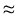1 : 3

Hence, option 3.

QUESTION: 16

Answer the following question based on the information given below.Cereal consumption of Maharashtra state in 000 ton

Q.Which month has highest cereal consumption in 2012?

Solution:

Consider the monthly cereal consumption ( in 000 tons) in 2012 for only the five months mentioned in the answer options.

Aug = 18.1 + 13.9 + 25.5 = 57.5

Sep = 17.4 + 13.8 + 23.4 = 54.6

Oct = 17.6 + 14.3 + 24.5 = 56.4

Nov = 17.8 + 15.3 + 23.6 = 56.7

Dec = 16.1 + 15.2 + 25.1 = 56.4

Hence August has the maximum cereal consumption in 2012.
Hence, option 5.

QUESTION: 17

Answer the following question based on the information given below.Cereal consumption of Maharashtra state in 000 ton

Q.Which of the following cases shows the maximum instances of a negative percentage change in the monthly consumption?

Solution:

A negative percentage change in the monthly consumption basically implies that the consumption in a particular month is less than the consumption in the previous month.
The number of times there is a negative percentage change for each option is:
Wheat in 2011 : 4 times - Mar, June, Sept, and Dec

Jwari in 2011 : 2 times - April and Oct Rice in 2012 : 5 times - Mar, April, Jun, Sept and Nov

Jwari in 2012 : 5 times - June, Jul, Aug, Sept and Dec

Thus, the rice consumption in 2012 as well as the jwari consumption in 2012 both show the most instances of a negative percentage change.
Hence, option 5.

QUESTION: 18

Answer the following question based on the information given below.Cereal consumption of Maharashtra state in 000 ton

Q.What is the maximum percentage change in the rice consumption for the year 2011?

Solution:

Here, observe that the absolute change in the rice consumption in 2011 was less than 2000 tons for every consecutive month apart from December.
Between November and December, the rice consumption increased by 2400 tons.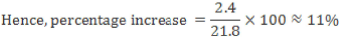It can be verified that the percentage change in all other months was less than this value.
Hence, option 1.

QUESTION: 19

There is a field which is in the shape of a quarter circle. A cow is tied on the field such that the circle formed by its grazing is exactly inscribed in the quarter. What is the ratio of area which is grazed and that which is not?

Solution:

The figure is as shown below.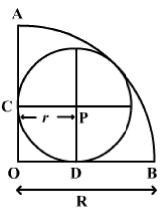The cow is grazing along the circle with centre as P while the field is a quarter with centre at O. Let the smaller circle touch the bigger circle along its circumference at Z.

Now, let the radius of bigger circle be R and that of smaller circle be r.
Now, CP = DP = r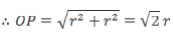Now, if we extend line OP to meet the circle at Z, then

QUESTION: 20

A toy is made by mounting a hemisphere of radius 7 cm over a right circular cylinder of the same radius. (Flat surface of hemisphere coincides with that of the right circular cylinder.) Height of the clinder is 10 cm. What will be the cost of painting the entire toy except the base, if the cost of painting is Rs. 14 per cm2?

Solution:

Note that we need to paint only curved surface areas of hemisphere and cylinder.
Curved Surface Area of the hemisphere = 2πr2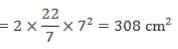Curved Surface Area of the right circular cylinder = 2πrh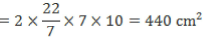Total surface area that is to be painted = (308 + 440) cm2 = 748 cm2

Cost of painting the toy = 748 x 14 = Rs. 10,472

Hence, option 3.

QUESTION: 21

Arun and Ram work together and complete the construction of a wall in x days. When Arun works alone, he takes 18 more days than x. When Ram works alone, he takes 8 more days than x. If they are paid an amount of Rs. 600 for the entire work in proportion to their work done, what is the difference between the amounts that Aran and Ram receive?

Solution:

Aran takes x + 18 days and Ram takes x + 8 days to complete the work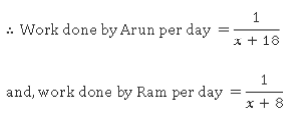Working together, they finish the work in x days.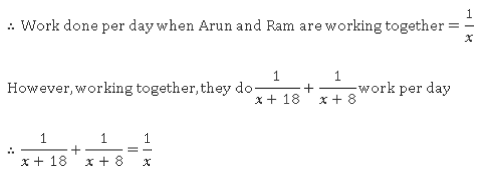Solving this equation, x = 12

Hence, working together, Arun and Ram complete the work in 12 days.
Individually, Aran takes 30 days and Ram takes 20 days.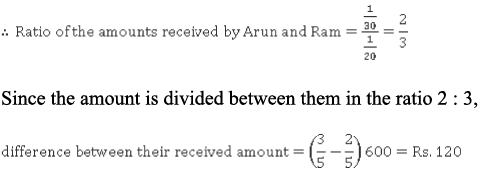Hence, option 2.

QUESTION: 22

In a triangle ABC, AD is the median to BC. If AB = BC = 12 cm and AC = 8 cm, AD = ?

Solution:

Since AD is the median to BC, BD - CD - BC/2 - 12/2 - 6 cm

According to the Apollonius’ Theorem, AB2 + AC2 = 2(AD2 + BD2)
122 + 82 = 2(AD2 + 62)
144 + 64 = 2 AD2 + 72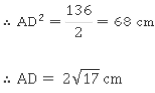Hence, option 4.

QUESTION: 23

2500 students gave an examination comprising five questions. Each question had five answer choices, of which only one was correct. The marking scheme was +4/-1 for every correct/incorrect answer. Each student attempted all the questions. If the overall answer key of no two students was the same, what is the minimum number of students with a positive score?

Solution:

Each student attempted all the questions and no two students had the same answer key.
Since each question had 5 options, each question could have been answered in 5 different ways.
Since there were 5 such questions, total possible answer keys = 55 = 3125, which exceeds the number of students (i.e. 2500).
Hence, only 2500 of the possible 3125 answer keys will be used.
Minimum number of students getting a positive score = Total number of students - Maximum number of students who can get a zero or a negative score
Since every correct and incorrect answer gives +4 and -1 marks respectively, a student should answer 1 question correctly and 4 questions incorrectly to get a score of 0.
The question that is answered correctly can be selected in 5 different ways.
For any question that is answered incorrectly, the student can select one out of the four incorrect options in 4 different ways.
Since there are four questions answered incorrectly, total ways to get a score of0 = 5 x44 = 5 x 256= 1280 Hence, maximum number of students who can get a score of 0 = 1280

To get a negative score, a student should answer all the questions incorrectly.

As explained above, one question can be incorrectly answered in 4 ways.
Since all 5 questions are incorrectly answered, total ways to get a negative score = 45 = 1024. This is the maximum number of students who can get a negative score.
The minimum number of students getting a net positive score = 2500 - (1280+ 1024) = 196

Hence, option 2.

QUESTION: 24

The total number of chocolates with Ram and Lakhan is more than 85 but less than 95. If Lakhan gives a particular number of chocolates to Ram, then Ram will have eight times the number of chocolates left with Lakhan and if Ram gives the same number of chocolates to Lakhan, then Lakhan will have double the number of chocolates left with Ram. How many chocolates does Ram have?

Solution:

The total number of chocolates has to lie between 85 and 95 and has to be divisible by 8+1=9 as well as 2+1=3. The only number satisfying these is 90.
Result I: Ram 80, Lakhan 10
Result II: Ram 30, Lakhan 60
Number of chocolates with Ram = (80+30)/2 = 55

QUESTION: 25

How many six-digit numbers are there such that the 3rd digit is the square of the 1st digit and the 4th digit is the square of the 2nd digit?

Solution:

Option (4).    1200 is the correct answer.

Explanation:-

We need to find the no. Which is of the form aba^2b^2cd ; where a,b,c and d can take any value from 0 to 9.

a and b cannot exceed 3 as the square of the two numbers then would exceed 9, which is not possible.

A can have 3 values ( 1,2 or 3) and b can have 4 values ( 0,1,2 or 3) c and d can have 10 values each.

Thus the total count of numbers that satisfy the given conditions is :- 3*4*10*10

=> 1200 es each.

Thus total count of numbers that satisfy the given conditions is 3 x 4 x 10 = 1200

QUESTION: 26

Group Question

Answer the following question based on the information given below.

The chart below shows the selling price (in Rs. 000s) of plywood, saw timber and logs. The price of logs is per cubic metre and while that of plywood and saw timber is per tonne. All prices are integral.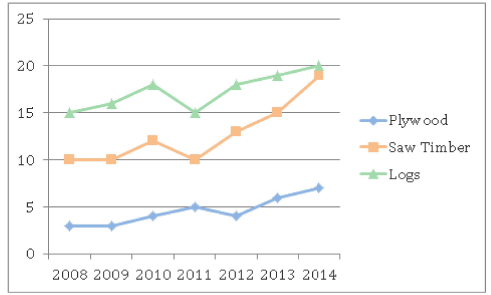1 cubic metre of plywood = 1 cubic metre of saw timber = 800 kg

Q.How many cubic metres of plywood would have to be sold in 2011 to yield the same revenue as generated from the sales of 220 tonnes of saw timber in 2014?

Solution:

Revenue generated from 220 tonnes of saw timber in 2014 = 220 x 19000 = Rs. 41.8 lakhs

Quantity of plywood sold (in tonnes) in 2011 to generate the same revenue = 4180000/5000 = 836
Since 800 kg of plywood corresponds to 1 cubic metre, (836 x 1000) kg of plywood corresponds to (836 x 1000)/800 cubic metre i.e. 1045 cubic metre

Hence, option 2.

QUESTION: 27

The chart below shows the selling price (in Rs. 000s) of plywood, saw timber and logs. The price of logs is per cubic metre and while that of plywood and saw timber is per tonne. All prices are integral.1 cubic metre of plywood = 1 cubic metre of saw timber = 800 kg

Q.In which year is the total selling price of 1 cubic metre of each of the three products the least?

Solution:

For plywood and saw timber, 1 cubic metre = 800 kg = 0.8 tonne

If the selling price of 1 tonne of plywood or saw timber is Rs. jc, the selling price of 0.8 tonnes is Rs. (0.8x) For any year, total selling price per tonne = logs + 0.8(plywood) + 0.8(saw timber) = logs + 0.8(plywood + saw timber)

Since one of the options is ‘none of the above’, calculate the total selling price for each year.
By observation, all three products have their least selling price in 2008. Hence, the overall least selling price is also in 2008.
Hence, option 5.

QUESTION: 28

The chart below shows the selling price (in Rs. 000s) of plywood, saw timber and logs. The price of logs is per cubic metre and while that of plywood and saw timber is per tonne. All prices are integral.1 cubic metre of plywood = 1 cubic metre of saw timber = 800 kg

Q.In 2010, what is the ratio of the selling price per tonne for plywood, logs and saw timber?

Solution:

The selling price of logs is given per cubic metre but the conversion from cubic metre to tonnes is not given for logs.
Hence, the selling price per tonne cannot be found for logs.
Hence, the required ratio cannot be found.
Hence, option 5.

QUESTION: 29

The chart below shows the selling price (in Rs. 000s) of plywood, saw timber and logs. The price of logs is per cubic metre and while that of plywood and saw timber is per tonne. All prices are integral.1 cubic metre of plywood = 1 cubic metre of saw timber = 800 kg

Q.In 2012, if plywood, saw timber and logs contributed to 20%, 30% and 50% of the total sales (by cubic metre), what was the average selling price (in Rs. 000s) per cubic metre?

Solution:

Consider the solution to the earlier questions.
If selling price of plywood or saw timber = Rs. x per tonne, then selling price = Rs. (0.8x) per cubic metre.

In 2012, selling price per cubic metre (in 000s) for all three products is:

logs =18, plywood = 0.8 x 4 = 3.2 and saw timber = 0.8 x 13 = 10.4

Now, let 100 cubic metres be sold in all.
Total selling price = (20 x 3.2) + (30 x 10.4) + (50 x 18) = 64 + 312 + 900 = 1276
Average selling price (in Rs. 000s) per cubic metre = 1276/100 = 12.7613

Hence, option 4.

QUESTION: 30

Group Question

Answer the following question based on the information given below.

The expenses of a particular household fall under 4 broad headings, as shown below.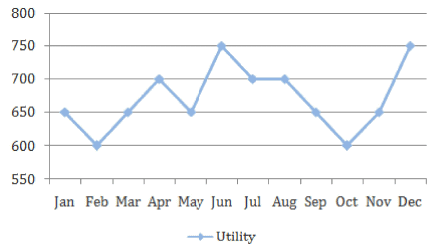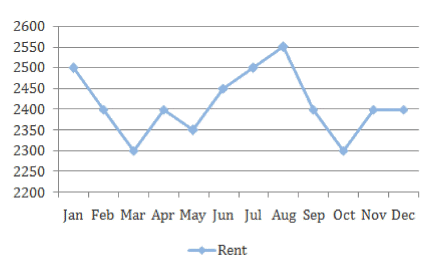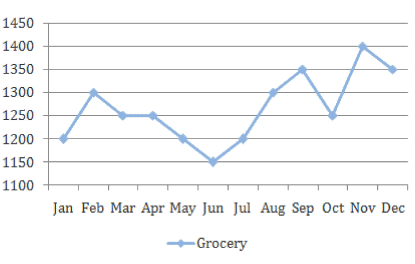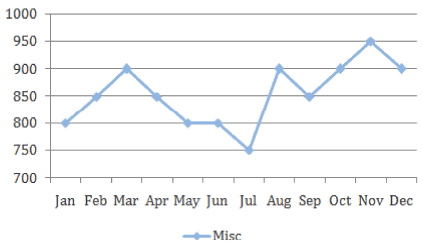The overall monthly expenditure of the household is the sum of the individual expenditure on each head in the month. All figures in the graphs are in actuals.
The income of this household is constant at Rs. 10,000 per month. Any unspent amount is saved in this household.

Q.In which month are the savings highest for the household?

Solution: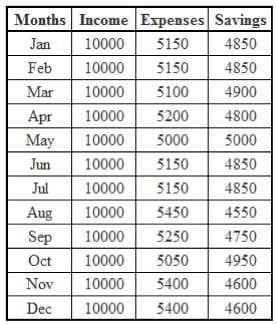Savings = Income - (Utility + Rent + Grocery + Misc)

The savings for each month are as shown in the table above.

Thus, the saving are highest in May.

Hence, option 3.

QUESTION: 31

The expenses of a particular household fall under 4 broad headings, as shown below.The overall monthly expenditure of the household is the sum of the individual expenditure on each head in the month. All figures in the graphs are in actuals.
The income of this household is constant at Rs. 10,000 per month. Any unspent amount is saved in this household.

Q.How many times have all the expenses increased together as compared to the previous month? Assume that the expenses in December of the previous year were same as January of this year.

Solution:

All the expenses have increased together only in the month of November i.e. only once.
Hence, option 2.

QUESTION: 32

The expenses of a particular household fall under 4 broad headings, as shown below.The overall monthly expenditure of the household is the sum of the individual expenditure on each head in the month. All figures in the graphs are in actuals.
The income of this household is constant at Rs. 10,000 per month. Any unspent amount is saved in this household.

Q.The overall household expenditure is said to be “under control” if at least 3 of the 4 expense heads do not increase in value from the previous month. In how many months have the household expenses been “under control”?

Solution:

Here, for each expense head, identify the months where the value is constant or smaller compared to the previous month.

Utility: Feb, May, Jul, Aug, Sep, Oct Rent: Feb, Mar, May, Sep, Oct, Dec Grocery: Mar, Apr, May, Jun, Oct, Dec Misc: Apr, May, Jun, Jul, Sep, Dec Thus,Sep, Oct and Dec appear thrice while May appears for all expense heads.
Thus, expenditure was “in control” in these four months.
Hence, option 4.

QUESTION: 33

The expenses of a particular household fall under 4 broad headings, as shown below.The overall monthly expenditure of the household is the sum of the individual expenditure on each head in the month. All figures in the graphs are in actuals.
The income of this household is constant at Rs. 10,000 per month. Any unspent amount is saved in this household.

Q.If the head of this household wants his average monthly savings to be 52% of his average m onthly income, by what percentage does he need to reduce his average monthly expenditure ?

Solution:

Total savings (in Rs.) = 4850 + 4850 + 4900 + 4800 + 5000 + 4850 + 4850 + 4550 + 4750 + 4950 + 4600 + 4600 = 57550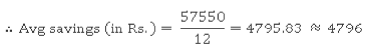The average monthly income is Rs. 10,000

Since the average saving shave to be come 52 % of the average monthly income, they should become Rs. 5,200 per month, and average monthly expenditure should become Rs. 4,800.
Current average monthly expenditure = 10000 - 4796 = Rs. 5204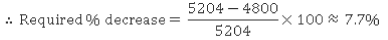Hence, option 3.

QUESTION: 34

If 2 sinA = 2 - cos2A, which of these can not be the value of A ( in °)?

Solution:

2sinA = 2 - cos2A

2sinA = 2 - (1 - sin24)

sin2A - 2sin A + 1 = 0

(simA - 1 )2 = 0

sinA = 1

Now, sin 0 = 0, sin 90 = 1 , sin 180 = 0, sin 270 = -1, sin 360 = 0 , sin 450 1 and so on.
Thus, sinA = 1 for all A = 90 + 360n, where n is a whole number.

All the values except 630° are of the form 90 + 360 n.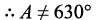Hence, option 2.

QUESTION: 35

A bank offers two short term schemes:

1. Deposit a sum for 2 years on compound interest at r % p.a.

2. Deposit a sum for 3 years on simple interest at R % p.a.

Q.If, for a particular sum (P), either scheme yields the same amount and R/r = 2, what is the value of R?

Solution:

According to the data given: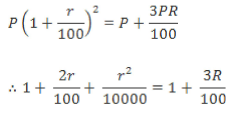R = 2r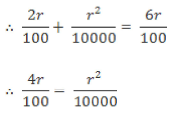r = 400

R = 400 x 22 = 800

Hence, option 3.

QUESTION: 36

Equation of a pair of line passing through the origin is 2x2 + xy - y2 = 0. What are the separate equations of the lines?

Solution:

2x2 + xy - y2 = 0 is a second degree equation that represents a pair of straight lines passing through the origin.

2x2 + 2xy -  xy -  y2 = 0

2x(x +y) - y(x + y) = 0

2x - y = 0 and x + y = 0 are the separate equations of the line.
Hence, option 4.

QUESTION: 37

In class A, the ratio of the average age of boys to the average age of girls is 1 : 2 and the ratio of number of boys to the number of girls is 2 : 1. In class B, the ratio of average age of boys to the average age of girls is 3 : 2 and the ratio of number of boys to the number of girls is 1 : 2. The number of students in class A and class B are equal. If the average age of class A is equal to the average age of class B, then what is the ratio of the average age of girls of class A to the average age of boys of class B?

Solution:

For class A:

Let the average age of boys be x and the number of girls be y.
Average age of girls is 2x and the number of boys is 2y.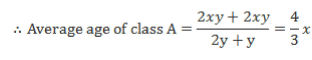For class B: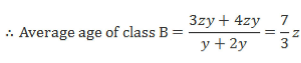Let ‘z’ be the common variable for average age of boys and girls.
Average age of boys is 3z and average age of girls is 2z.
Since, the total number of students is equal in both classes, the number of boys is y and the number of girls is 2y.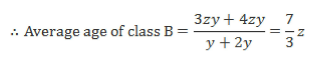Now, since the average ages of both classes are equal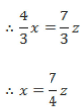Ratio of average age of girls of class A to the average age of boys of class B is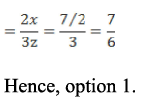QUESTION: 38

Three fair dice are rolled. What is the probability that the sum of scores obtained is 12?

Solution:

The number of different scores that can be obtained = 63 = 216
The number of ways in which a score of 12 can be obtained is given in the following table.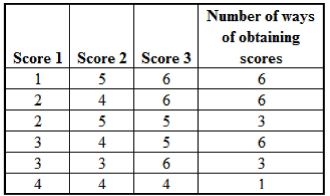The total number of ways of obtaining a score of 12 is 25.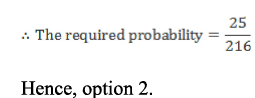QUESTION: 39

A, B and C are to ride cars on a circular track of length 60 m such that only people drive together at a given time, in opposite directions. When A and B drive, they meet for the first time after 12 seconds. Similarly, B and C meet for the first time after 15 seconds while A and C meet for the first time after 20 seconds. What is the sum of their individual speeds?

Solution:

Let the individual speed of A, B and C (in m/s) be a, b and c respectively.
Since any pair of two drivers rides in opposite directions, their relative speed is the sum of their individual speeds.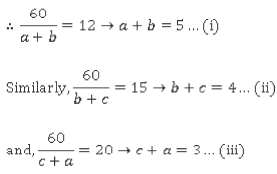Adding the three equations,

2 (a + b + c) = 5 + 4 + 3 = 12

Sum of individual speeds = a + b + c = 6

Hence, option 3.

QUESTION: 40

Affoz travels 25% faster than Amir. Both start from Surat at the same time and reach Ahmedabad, which is 250 kms away, at the same time using the same route. On the way, however, Affoz lost 50 minutes while stopping at various restaurants. What is Amir’s speed?

Solution:

Let the speed of Amir be x km/hr Speed of Afroz = 1.25x km/hr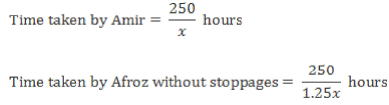Afroz lost 50 minutes due to stoppages.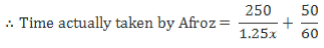Amir and Afroz reached Ahmedabad at the same time.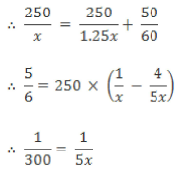x = 60 km/hr

Hence, option 2.

QUESTION: 41

In a quiz contest, the host shakes hands with each participant twice - at the start of the contest and then, at the end. Besides, he shakes hands with each finalist (selected from amongst the participants) three more times. The participants are also required to shake hands with each other once. If there are 11 participants in all and 3 of them become finalists, what is the total number of handshakes?

Solution:

The host shakes hands with 11 participants twice i.e. 11 x 2 = 22 handshakes

The host also shakes hands with the 3 finalists thrice i.e. 3 x 3 = 9 handshakes
Finally, the 11 participants shake hands with each other once i.e.  11C2 = 55 handshakes

Total handshakes = 22+ 9 + 55 = 86 Hence, option 5.

QUESTION: 42

The 15th term of the equation (5a + b)16 is:

Solution:

In the expansion of (x + y)n, we can find the (k + 1)th term using binomial expansion.
We can find the (k + 1)th term using the formula given below: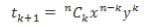the 15th term = t14 + 1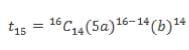t15 = 120 x (5a)2 x b14

t15= 3000a2b14

Hence, option 3.

QUESTION: 43

Each question is followed by two statements, A and B. Answer each question using the following instructions:

Mark option (1) if the question can be answered by using statement A alone but not by using statement B alone.
Mark option (2) if the question can be answered by using statement B alone but not by using statement A alone.
Mark option (3) if the question can be answered by using either statement alone.
Mark option (4) if the question can be answered by using both the statements together but not by either of the statements alone.
Mark option (5) if the question cannot be answered on the basis of the two statements.

Q.What is the tenth term of the given arithmetic series?

A. The first term of the arithmetic series is 17.
B. The sum of the fourth term and the sixteenth term of the arithmetic series is 142.

Solution:

Let the first term and common difference of the given arithmetic series be a and d respectively.

Using statement A alone:

The first term of the series is 17.

a =17

We need to find the tenth term i.e. a + 9d.
Since we cannot find the value of d from the above information, we cannot find the value of the tenth term.
Thus, the question cannot be answered using statement A alone.
Using statement B alone:

The sum of the fourth term and the sixteenth term of the given series is 142.

a + 3d + a + 15d = 142

i.e. 2a+ 18d= 142

i.e. a + 9d = 71

Thus, the tenth term of the given series is 71.
Thus, the question can be answered using statement B alone.
Thus, the question can be answered using statement B alone but not by using statement A alone.
Hence, option 2.

QUESTION: 44

Each question is followed by two statements, A and B. Answer each question using the following instructions:

Mark option (1) if the question can be answered by using statement A alone but not by using statement B alone.
Mark option (2) if the question can be answered by using statement B alone but not by using statement A alone.
Mark option (3) if the question can be answered by using either statement alone.
Mark option (4) if the question can be answered by using both the statements together but not by either of the statements alone.
Mark option (5) if the question cannot be answered on the basis of the two statements.

Q.In a given year, Suresh has to take 100 tests. Each test carries a maximum of 100 marks, and there are no negative marks in the tests. Suresh has set himself a target of achieving at least 75% aggregate marks. In the first 40 tests Suresh achieved an aggregate of 60% marks. How many tests remained to be taken when Suresh achieved his overall target?

A. Suresh had an aggregate of 84% marks in 100 tests.
B. Suresh had an aggregate of 80% marks in 98 tests.

Solution:

Maximum marks that Suresh could have achieved = 100(100) = 10000 Suresh’s target = 100(75) = 7500

In the first 40 tests, Suresh achieved an aggregate of 60% marks i.e. 40(60) = 2400 marks

He now needs 7500 - 2400 = 5100 marks to achieve his target.
Using Statement A alone:

Suresh achieved an aggregate of 84% marks in 100 tests i.e. he got 8400 marks in 100 tests.
Out of these, he had got 2400 marks in 40 tests.
Hence, he got 8400 - 2400 = 6000 marks in the remaining 60 tests.
This implies that he got 100 marks in each of his remaining tests.
Thus, he would have achieved his target after the next 51 tests i.e. with 9 tests remaining.
Thus, the question can be answered using statement A alone.
Using Statement B alone:

Suresh achieved an aggregate of 80% marks in 98 tests i.e. he got 8000 marks in 98 tests.
Out of these, he had got 2400 marks in 40 tests.
Hence, he got 8000 - 2400 = 5600 marks in the remaining 58 tests.
Here, the marks per test cannot be found and hence it cannot be found as to when Suresh achieved his target.
Thus, the question cannot be answered using statement B alone.
Thus, the question can be answered using statement A alone but not by using statement B alone.
Hence, option 1.

QUESTION: 45

Each question is followed by two statements, A and B. Answer each question using the following instructions:

Mark option (1) if the question can be answered by using statement A alone but not by using statement B alone.
Mark option (2) if the question can be answered by using statement B alone but not by using statement A alone.
Mark option (3) if the question can be answered by using either statement alone.
Mark option (4) if the question can be answered by using both the statements together but not by either of the statements alone.
Mark option (5) if the question cannot be answered on the basis of the two statements.

Q.What is the area of triangle BCF formed inside a rectangle ABCD such that F lies on AD?

A. The sides of the rectangle measure 6 cm and 8 cm.
B. The area of the rectangle is equal to the area of a square of side 6 cm.

Solution:

A possible representation of triangle BCF and rectangle ABCD is shown below: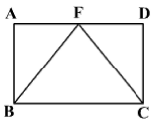A triangle having the same base as a rectangle and between same parallels has half the area of the rectangle.
Thus, the area of ΔBCF is half the area of the rectangle.
Using statement A alone:

Since the sides of the rectangle measure 6 cm and 8 cm, the area of the rectangle is 48 sq.cm.
So, the area of the triangle is 24 sq.cm.
Thus, the question can be answered using statement A alone.
Using statement B alone:

The area of the rectangle is the same as that of a square of side 6 cm i.e. 36 sq.cm
So, the area of the triangle is 18 sq.cm.
Thus, the question can be answered using statement B alone.
Thus, the question can be answered using either statement alone.
Hence, option 3.

QUESTION: 46

Each question is followed by two statements, I and II. Answer each question using the following instructions:

Mark (1) if the question can be answered by using statement I alone but not by using statement II alone.
Mark (2) if the question can be answered by using statement II alone but not by using statement I alone.

Mark (3) if the question can be answered by using either of the statements alone.
Mark (4) if the question can be answered by using both the statements together but not by either of the statements alone.
Mark (5)  if the question cannot be answered on the basis of the two statements.

Q.if the question cannot be answered by using any of the statements. x is a real number. Is x < 0?

I. (x - y)3 < 0

II. x2y3< 0

Solution:

Using statement I alone:

(x - y)3 < 0 ⇒ x - y < 0 ⇒ x < y

We cannot determine whether the value of x is negative.
Using statement II alone:

x2 is always positive and x2y3 is negative.
⇒ y< 0

We cannot determine whether the value of x is negative.
Using both the statements together:

x < y and y < 0 ⇒ x < 0

Thus, the question can be answered using both the statements together but not by either of the statements alone.
Hence, option 4.

QUESTION: 47

The question is followed by two statements, A and B. Answer the question using the following instructions:

Mark option (1) if the question can be answered by using statement A alone but not by using statement B alone.
Mark option (2) if the question can be answered by using statement B alone but not by using statement A alone.
Mark option (3) if the question can be answered by using either statement alone.
Mark option (4) if the question can be answered by using both the statements together but not by either of the statements alone.
Mark option (5) if the question cannot be answered on the basis of the two statements.

Q.If 3q = 7.5p + 4.5r, p : q : r = ?

A. 5p - 2q + 3r = 0

B. 4q - 8r + 5p = 0

Solution:

3q = 1.5p + 4.5r ... (1)

Divide both sides by r.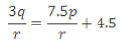Since the value of q/r and p/r is not known,p : q : r cannot be found.
Using statement A alone:

5p - 2q + 3r = 0

2q = 5p + 3r Multiplying both sides by 1.5.

3q = 7.5p + 4.5r
This is the same as the equation given in the question.
So, the required ratios cannot be found.

Thus, the question cannot be answered using statement A alone. Using statement B alone:

4q - 8r + 5p = 0

8r = 4q + 5p ... (2)

From (1), 3 q = 1.5p + 4.5r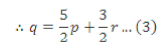Substitute the value of q in (2).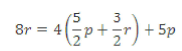8r = 10p + 6r + 5p

2r = 15p

p/r = 2/15
Once p/r is known, the value of qlr can be found from the original equation.
Once p : r and q : r are known, p : q : r can be easily found.
Thus, the question can be answered using statement B alone.
Thus, the question can be answered using statement B alone, but not by using statement A alone.
Hence, option 2.

QUESTION: 48

The question is followed by two statements, A and B. Answer the question using the following instructions:

Mark option (1) if the question can be answered by using statement A alone but not by using statement B alone.
Mark option (2) if the question can be answered by using statement B alone but not by using statement A alone.
Mark option (3) if the question can be answered by using either statement alone.
Mark option (4) if the question can be answered by using both the statements together but not by either of the statements alone.
Mark option (5) if the question cannot be answered on the basis of the two statements.

Q.What is the first term of an arithmetic progression consisting of positive integers only?
A. Sum of the squares of the second and the third term is 130.
B. The sixth term is divisible by three.

Solution:

Using statement A alone:

Sum of squares of only two pair of positive integers (i.e. (7, 9) and (3, 11)) is equal to 130.
So the second and third terms can be (7, 9), (9, 7) or (11, 3).

(3, 11) is not possible, as in that case the first term of the AP will become negative.
But still we cannot find exact value of second and third term of an AP. Hence, no conclusion can be drawn about the first term of an AP.
Hence, statement A alone is not sufficient to answer the question.
Using statement B alone: This does not provide any useful information about the first term of the AP. Hence, statement B alone is not sufficient to answer the question.
Using both the statements together:

From statement A it is clear that the second and third terms can be (7, 9), (9, 7) or (11, 3).
The different possible APs are

5, 7, 9, 11, 13, 15,... (possible)

11, 9, 7, 5, 3, 1 (sixth term is not divisible by 3, not possible)

19, 11, 3, -5, -13, -21 (sixth term is not positive, not possible)

Hence, the first term of the AP will be 5.
Hence, question can be answered using both the statements together.
Hence, option 4.

(9, 7) and (11,3) does have negative common difference. But that doesn't mean that these AP will be negative at some point. Because we don't know the number of elements in these series we can't assume them as infinte series or finite series.
AP could be,

11,9, 7 ,..., 1 and 19, 11,3.Use Code STAYHOME200 and get INR 200 additional OFF Use Coupon Code

### How to Prepare for CAT

Read our guide to prepare for CAT which is created by Toppers & the best Teachers